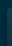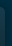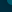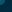# Beatles - Let It Be Chords & Tabs## Let It Be Chords & Tabs

Version: 9 Type: Chords
`Let It Be Chords`

```               LET IT BE - THE BEATLES
Chords by: Nischal "neesh"
Intro: D A Bm G
D A G D

VERSE 1:
D                     A                Bm               G
When I find myself in times of trouble, Mother Mary comes to me
D                 A              G  D
Speaking words of wisdom, let it be

D                A                Bm                 G
And in my hour of darkness, She is standing right in front of me
D                 A              G  D
Speaking words of wisdom, let it be

CHORUS:
D                  Bm         G          D
Let it be, let it be, let it be, let it be
D                A              G  D
Whisper words of wisdom, let it be

VERSE 2:
D                  A               Bm               G
When all the broken hearted people, Living in the world agree
D                A              G  D
There will be an answer, let it be

D                        A             Bm                       G
But though they may be parted, There is still a chance that they will see
D                A              G  D
There will be an answer, let it be
[ Tab from: https://www.guitartabs.cc/tabs/b/beatles/let_it_be_crd_ver_9.html ]
CHORUS:
D                  Bm         G          D
Let it be, let it be, let it be, let it be
D                A              G  D
There will be an answer, let it be
D                  Bm         G          D
Let it be, let it be, let it be, let it be
D                A              G  D
Whisper words of wisdom, let it be
D                  Bm         G          D
Let it be, let it be, let it be, let it be
D              A              G  D
There will be answer, let it be

SOLO

CHORUS:
D                  Bm         G          D
Let it be, let it be, let it be, let it be
D              A              G  D
There will be answer, let it be

VERSE 3:
D                     A
And when the night is cloudy,
Bm                       G
There is still a light that shines on me
D                 A             G  D
Shine on till tomorrow, let it be
D                         A      Bm              G
I wake up to the sound of music, Mother Mary comes to me
D                 A              G  D
There will be no sorrow, let it be

CHORUS:
D                  Bm         G          D
Let it be, let it be, let it be, let it be
D                 A              G  D
There will be no sorrow, let it be
D                  Bm         G          D
Let it be, let it be, let it be, let it be
D                A              G  D
Whisper words of wisdom, let it be```